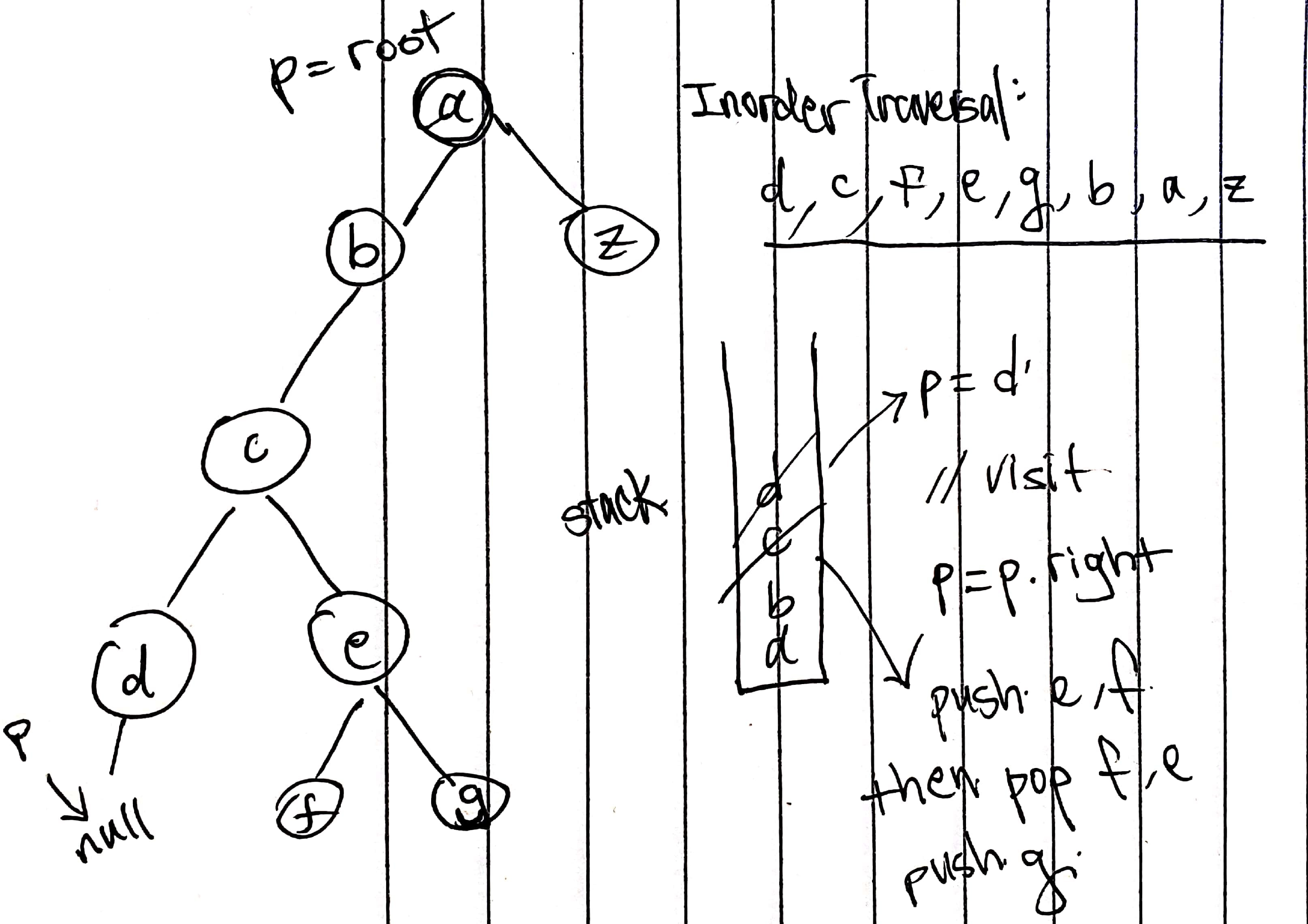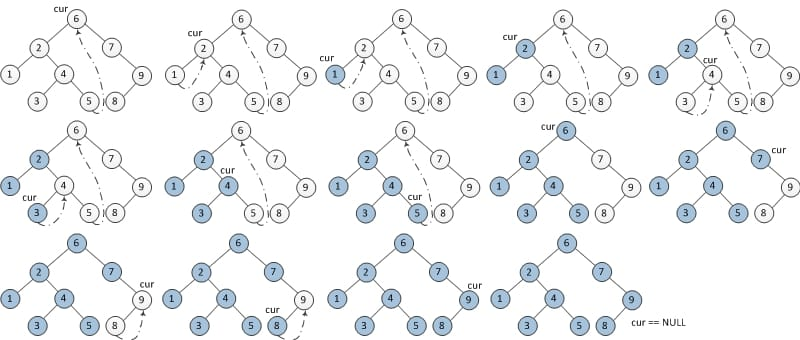# 94. Binary Tree Inorder Traversal

Reference: LeetCode
Difficulty: Medium

## Problem

Given a binary tree, return the inorder traversal of its nodes’ values.

Follow up: Recursive solution is trivial, could you do it iteratively?

## Analysis

### Recursion

Time: $O(N)$ since the recursive function is $T(N) = 2T(N/2) + 1$
Space: $O(\log{N})$ (when balanced); $O(N)$ (worst).

### Iteration

Note:

• The condition is p != null || !stack.isEmpty().
• Notice p = p.right:
• If p.right is null, the next node would be the node currently in the stack.
• Otherwise, the next node would be the leftmost node of the right child (after continuous pushing).“while” version:

“if” version:

Time: $O(N)$
Space: $O(\log{N})$ (when balanced); $O(N)$ (worst).

### Morris Traversal

Morris Traversal (reference)

Basic Idea: Morris Traversal uses the idea of threaded binary tree. It uses the left and right pointers of leaves to access the predecessor and successor in a specific traversal.

Step: (p is the current node)

1. If p‘s left child is null, output p and set its right child as the new p.
2. If p‘s left child is not null, then we find the predecessor of p in the left subtree.
1. If the predecessor‘s right child is null, set its right child to p, and update p with its left child.
2. If the predecessor‘s right child is p, set its right child to null (restore), and output p and update p with its right child.
3. Repeat the steps above until p is null.Time: $O(N)$

Intuitively, we may think the time complexity is $O(N\log{N})$ since finding a predecessor of a node takes $O(\log{N})$. In fact, finding all predecessors of all nodes just costs $O(N)$ time because in the whole process each edge/link is only traversed twice, the first time is to locate a node and the second time is to find the predecessor.

Space: $O(1)$

CommentJunhao Wang
a software engineering cat